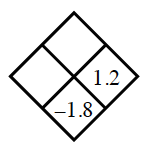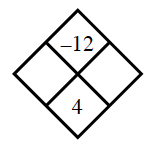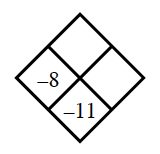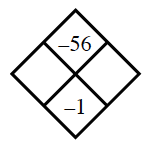### Home > CC2 > Chapter 4 > Lesson 4.1.2 > Problem4-19

4-19.

Copy and complete each of the Diamond Problems below. The pattern used in the Diamond Problems is shown at right.1.What number plus $1.2$ equals $-1.8$?

Multiply your answer by $1.2$ to find the top portion of the diamond.

Right: $(-1.8)-1.2=-3$
Top: $1.2(-3)=-3.6$

1.What two numbers add to $4$ and, when multiplied together, equal $-12$?

1.See part (a).

1.See part (b).

$7$ and $-8$